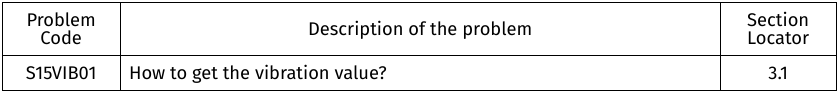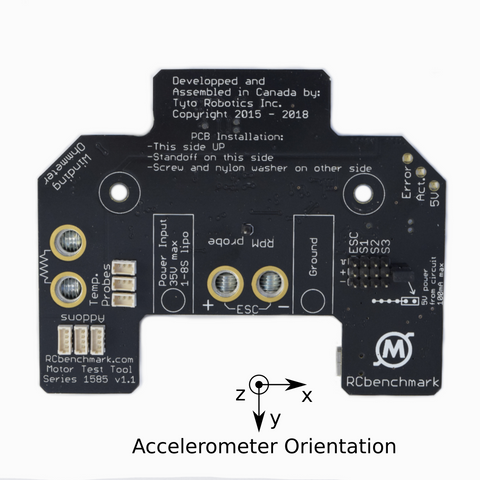-
| /
Save up to %
Save %
Save up to
Save
Sale
Sold out
In stock
Search

# Vibration Calculation & Accelerometer Setup

## ​1​. Applicable products

• RCbenchmark Series 1580 Thrust Stand and Dynamometer
• RCbenchmark Series 1585 Thrust Stand and Dynamometer

## 2.​ What describes your problem?

Please find within the following table which describes your problem. Once selected, mark down the troubleshooting points to follow.## 3. Problem specific troubleshooting

### 3.1​ How to get the vibration value

The "g" calculation is based on the vibration level felt on the circuit board, which includes resonance on the thrust stand. For this reason, the measurement is just an approximation and should only be used for comparison purposes.

The accelerometer was added for two reasons: safety and relative measurement of imbalance. The acceleration measurement is a low pass version of the magnitude of the (acceleration vector minus the gravity vector). The accelerometer can be used to shut down the motor if the vibration is too high during a long scripted endurance test for example.

For the axis: Place the ESC in front of you so you can read "RCbenchmark.com Motor Test Tool".

1) The X axis is pointing right.

2) The Y axis is pointing down

3) The Z axis is pointing toward you.

The convention is left hand rule.

Here is the source code that calculates the vibration value from the accelerometer readings. In the code, the accx, accy, and accz variables represent the instantaneous acceleration in g.

float calcVibration(float accx, float accy, float accz){

static float accxlpf = 0;

static float accylpf = 0;

static float acczlpf = 0;

static float vibration = 0;

static byte init = false;

if(!init){

accxlpf = accx;

accylpf = accy;

acczlpf = accz;

init = true;

}

//Acc high pass filter

float accxhpf = accx-accxlpf;

float accyhpf = accy-accylpf;

float acczhpf = accz-acczlpf;

//Instant amplitude deviation

float amplitude = sqrt(accxhpf*accxhpf + accyhpf*accyhpf + acczhpf*acczhpf);

//Vibration filter with different decay/gain factors

#define FILTER 0.9925

vibration = FILTER * vibration + (1.0-FILTER) * amplitude;

return vibration;

}

### 3.2 What is the accelerometer orientation?

Please see the image below. The accelerometer unit in the exported csv file in g.